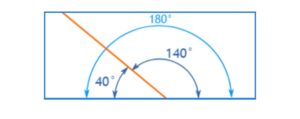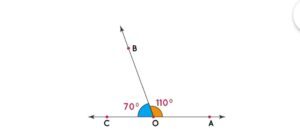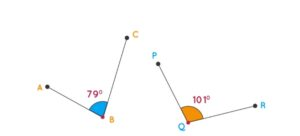Online Tution   »   Class 10   »   Supplementary Angels

# Supplementary Angels, Types, Example, Supplementary vs Complementary

## Supplementary Angels: What are Supplementary Angels?

In geometry, two angles are referred to as ‘complementary’ if the sum of their angles is 180°. Additionally, two angles are said to be supplementary when they add up to 180 degrees, and thus form a straight line together.

The symbol used to represent complementary angles is ‘s’. Complementary angles that make a sum of 180° create a right angle. More specifically, angle 1 and angle 2 can be identified as complementary when the equation angle 1 + angle 2 = 180° holds true. In this case, it is said that these two angles complement each other.Types of Angles in Maths, with Definition, Degrees

## Supplementary Angels Types

There are two types of Supplementary Angels as follow –

Adjacent Supplementary Angles are two angles that share a common vertex and arm, and possess a supplementary relationship; in other words, the combined measure of these angles is 180 degrees.For Example –

COB and AOB form a straight angle when they sum to 180 degrees (COB + AOB = 70° + 110° = 180°). These two angles are adjacent supplementary angles due to their shared vertex and common arm.

Non-adjacent Supplementary Angles are two supplementary angles that do not share the same vertex or line segment. These angles add up to make up a total of 180 degrees. When non-adjacent supplementary angles occur, they must face away from each other and will not be on the same side of the line or vertex.

For Example –The angles ABC and PQR are non-adjacent supplementary angles, as they neither share a common vertex or arm, yet they add up to 180° (i.e. 79° + 101° = 180°).

Thus, when these two non-adjacent supplementary angles are combined, the result is a straight angle.## Supplementary Angles: How to Find Supplementary Angles?

We can find the supplement of an angle in two ways: by using the properties of supplementary angles or by using the properties of complementary angles.

The supplement of an angle can be found using the properties of supplementary angles. If we know the measure of one angle in a pair of supplementary angles, we can find the measure of the other angle. This is because the sum of the angles is always 180 degrees.

We can also find the supplement of an angle by using the properties of complementary angles. We can find the complement of an angle by subtracting the measure of the given angle from 90.

Here is another way to find supplementary angels.

To find supplementary angles, we need to use the following formula:

s = 180 – a

where ‘a’ is the angle of interest.

For example – if we are given the angle measure of 30 degrees, we can use the formula to find its supplementary angle.

s = 180 – 30

s = 150 degrees

Therefore, the supplementary angle of 30 degrees is 150 degrees.

It is important to note that supplementary angles need not be adjacent to each other. However, they will always form a straight line when placed together.

Supplementary Angels, Types, Example , Supplementary vs Complementary

## Complementary Angles: Difference between Complementary and Supplementary Angles

In geometry, we read about two types of angles, which include complementary angle and supplementary angle, but often people get confused between these two angles and do not understand the difference between them, that’s why here we have complementary angle and supplementary angle. We are going to know the difference between so that we can understand the difference between these two angles.

 Complementary Angles Supplementary Angels if their sum is equal to 90 degrees, it is called Complementary Angels. if their sum is equal to 180 degrees, it is called Supplementary Angels. Complementary angles are typically used when solving problems that involve right triangles. This is because, in a right triangle, the two acute angles will always sum up to 90 degrees. Supplementary angles find their use in problems that involve straight lines or line segments. This is because, when two lines intersect to form a straight angle, the angle formed will always be 180 degrees. Complementary angles are only used to show right angles. Supplementary angles are used to show the mean of straight angles. Complementary angles are two angles that add up to 90 degrees and do not form a line when placed together. Supplementary angles are two angles that add up to 180 degrees and do form a line when placed together.## Tricks to Identify Supplementary & Complementary angles

Here we are telling you a trick through which you can easily understand and identify supplementary angles and complementary angles.

• It’s easy to remember that two Supplementary angles form a Straight angle by using the acronym “S for Straight.” When you think of this, think of when two Supplements are combined to form one complete Straight angle.
• The letter ‘C’ is an acronym for the concept of Complementary Angles. When adjacent C-angles are placed together, they form a right angle or corner. Memorizing this information can help students better understand and apply geometric principles in Math and beyond.

Sharing is caring!

## FAQs

### What are two supplementary angles?

The two types of supplementary angles are Adjacent Supplementary and Non-Adjacent Supplementary.

### Can 3 angles be supplementary?

No, because the definition of supplementary angle is two angles are said to be supplementary when they add up to 180 degrees.